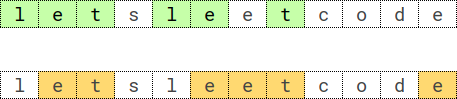Formatted question description: https://leetcode.ca/all/2014.html

# 2014. Longest Subsequence Repeated k Times (Hard)

You are given a string s of length n, and an integer k. You are tasked to find the longest subsequence repeated k times in string s.

A subsequence is a string that can be derived from another string by deleting some or no characters without changing the order of the remaining characters.

A subsequence seq is repeated k times in the string s if seq * k is a subsequence of s, where seq * k represents a string constructed by concatenating seq k times.

• For example, "bba" is repeated 2 times in the string "bababcba", because the string "bbabba", constructed by concatenating "bba" 2 times, is a subsequence of the string "bababcba".

Return the longest subsequence repeated k times in string s. If multiple such subsequences are found, return the lexicographically largest one. If there is no such subsequence, return an empty string.

Example 1:Input: s = "letsleetcode", k = 2
Output: "let"
Explanation: There are two longest subsequences repeated 2 times: "let" and "ete".
"let" is the lexicographically largest one.


Example 2:

Input: s = "bb", k = 2
Output: "b"
Explanation: The longest subsequence repeated 2 times is "b".


Example 3:

Input: s = "ab", k = 2
Output: ""
Explanation: There is no subsequence repeated 2 times. Empty string is returned.


Example 4:

Input: s = "bbabbabbbbabaababab", k = 3
Output: "bbbb"
Explanation: The longest subsequence "bbbb" is repeated 3 times in "bbabbabbbbabaababab".


Constraints:

• n == s.length
• 2 <= n, k <= 2000
• 2 <= n < k * 8
• s consists of lowercase English letters.

Companies:

Related Topics:
String, Backtracking, Greedy, Counting, Enumeration

Similar Questions:

## Solution 1. DFS

// OJ: https://leetcode.com/problems/longest-subsequence-repeated-k-times/
// Time: O(26^(N/K) * N)
// Space: O(N/K)
class Solution {
public:
string longestSubsequenceRepeatedK(string s, int k) {
int cnt = {};
string ans;
auto isSubsequence = [&]() {
int matched = 0, i = 0, j = 0, M = s.size(), N = ans.size();
for (; i < M && matched < k; ++i) {
if (s[i] == ans[j]) ++j;
if (j == N) j = 0, matched++;
}
return matched == k;
};
function<bool(int)> dfs = [&](int len) {
if (ans.size() == len) return true;
for (int i = 25; i >= 0; --i) {
if (cnt[i] == 0) continue;
ans.push_back('a' + i);
if (isSubsequence() && dfs(len)) return true;
ans.pop_back();
}
return false;
};
for (char c : s) cnt[c - 'a']++;
for (int &n : cnt) n /= k;
int j = 0;
for (int i = 0; i < s.size(); ++i) { // remove the characters in s that doesn't occurrs k times.
if (cnt[s[i] - 'a']) s[j++] = s[i];
}
s.resize(j);
for (int len = 7; len >= 1; --len) {
if (dfs(len)) return ans;
}
return "";
}
};


Or

// OJ: https://leetcode.com/problems/longest-subsequence-repeated-k-times/
// Time: O(26^(N/K) * N)
// Space: O(N/K)
class Solution {
string ans;
int cnt = {};
bool match(string &s, int k) {
int i = 0, j = 0, M = s.size(), N = ans.size();
for (; i < M && k; ++i) {
if (s[i] != ans[j]) continue;
++j;
if (j == N) j = 0, --k;
}
return k == 0;
}
bool dfs(string &s, int k, int len) {
if (len == 0) return true;
for (char c = 'z'; c >= 'a'; --c) {
if (ans.size() && ans.back() == c) continue;
int used = min(len, cnt[c - 'a']);
for (int i = 0; i < used; ++i) ans.push_back(c);
while (ans.size() && ans.back() == c) {
if (match(s, k) && dfs(s, k, len - used)) return true;
--used;
ans.pop_back();
}
}
return false;
}
public:
string longestSubsequenceRepeatedK(string s, int k) {
for (char c : s) cnt[c - 'a']++;
for (int &n : cnt) n /= k;
for (int len = s.size() / k; len >= 1; --len) {
if (dfs(s, k, len)) return ans;
}
return "";
}
};


## Solution 2. BFS

// OJ: https://leetcode.com/problems/longest-subsequence-repeated-k-times/
// Time: O(?)
// Space: O(?)
// Ref: https://leetcode.com/problems/longest-subsequence-repeated-k-times/discuss/1472755/short-solutionApriori-algorithm-and-BFS-beat-100
class Solution {
public:
string longestSubsequenceRepeatedK(string s, int k) {
queue<string> q{ {""} };
unordered_set<string> valid{""};
string ans;
while (q.size()) {
auto x = q.front();
q.pop();
for (char c = 'a'; c <= 'z'; ++c) {
auto y = x + c;
auto suffix = y.substr(1);
if (valid.count(suffix) == 0) continue; // suffix must be also valid.
int matched = 0, i = 0, j = 0;
for (; i < s.size() && matched < k; ++i) {
if (s[i] == y[j]) ++j;
if (j == y.size()) j = 0, ++matched;
}
if (matched == k) {
ans = y;
q.push(y);
valid.insert(y);
}
}
}
return ans;
}
};